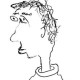# When is it supercuspidal?

Today I want to talk about an amazing theorem of Moeglin, which I learned from WTG.

Let$F/\mathbf{Q}_p$ be a finite extension,$G=\mathrm{SO}_{2n+1}$ the split odd special orthogonal group over$F$,$G'$ its unique inner form. By work of Arthur and Moeglin, there is a natural bijection between discrete series representations of$G$ or$G'$, and pairs$(\phi,\chi)$ where$\phi: W_F \times \mathrm{SL}_2 \to \mathrm{Sp}_{2n}$ is a discrete L-parameter and$\chi$ is a character of the centralizer group$A_\phi$. In this setting,$\phi$ is discrete if it is the sum of$m$ pairwise-distinct irreducible representations$\phi_i = \sigma_i \boxtimes [d_i] : W_F \times \mathrm{SL}_2 \to \mathrm{Sp}_{2n_i}$ with$\sum_{1 \leq i \leq m} n_i = n$. Here$[d]:\mathrm{SL}_2 \to \mathrm{SL}_d$ is the usual d-1st symmetric power representation. The associated centralizer group$A_\phi$ is of the form$\{ \pm 1\}^m$, and has a canonical basis indexed by the irreducible summands$\phi_i$. Given$(\phi,\chi)$, let$\pi(\phi,\chi)$ be the associated discrete series representation. Note that$\pi(\phi,\chi)$ is a representation of$G$ if$\chi$ is trivial on the evident subgroup$\{ \pm 1 \} = Z(\mathrm{Sp}_{2n}) \subset A_\phi$, and is a representation of$G'$ otherwise. This splits the representations up evenly: for$\phi$ fixed, there are$2^m$ possible$\chi$‘s, and we get a Vogan L-packet$\Pi_\phi = \Pi_\phi(G) \cup \Pi_\phi(G')$ where$\Pi_\phi(G)$ and$\Pi_\phi(G')$ each contain$2^{m-1}$ elements.

Question. When is$\pi(\phi,\chi)$ a supercuspidal representation?

For$n=2$ I had previously memorized the (already complicated) answer to this question, so you can imagine my pleasure when I learned that Moeglin found a simple conceptual criterion which works in general! To state her theorem, we need a little vocabulary.

Definition. A discrete parameter$\phi=\oplus_i \phi_i$ is without gaps if for every$\sigma \boxtimes [d]$ occurring among the$\phi_i$‘s with$d \geq 3$, then also$\sigma \boxtimes [d-2]$ occurs among the$\phi_i$‘s.

Definition. Suppose$\phi$ is without gaps. A character$\chi$ of the component group is alternating if for every pair$\sigma \boxtimes [d]$ and$\sigma \boxtimes [d-2]$ (with$d \geq 3$) occurring among the$\phi_i$‘s,$\chi(\sigma \boxtimes [d]) = - \chi (\sigma \boxtimes [d-2])$. Moreover we require that on every summand of the form$\sigma \boxtimes $, we have$\chi(\sigma \boxtimes )=-1.$

Theorem (Moeglin). The representation$\pi(\phi,\chi)$ is supercuspidal iff$\phi$ is without gaps and$\chi$ is alternating.

Example 0. By definition,$\phi$ is supercuspidal if$d_i =1$ for all summands. In this case,$\phi$ is (vacuously) without gaps and every$\chi$ is (vacuously) alernating, so$\Pi_\phi$ consists entirely of supercuspidal representations. The converse – if$\Pi_\phi$ consists only of supercuspidals then necessarily$\phi$ is supercuspidal – is also immediate!

Example 1. Let$\sigma_2, \sigma_2':W_F \to \mathrm{SL}_2$ be distinct supercuspidal parameters. Then$\phi = \sigma_2 \oplus \sigma_2' \oplus \sigma_2' \boxtimes :W_F \times \mathrm{SL}_2 \to \mathrm{Sp}_{10}$ is a discrete parameter without gaps, with component group of size 8. It is easy to see that four of the possible$\chi$‘s are alternating, and two of these are trivial on the center of$\mathrm{Sp}_{10}$. Thus, the packets$\Pi_\phi(\mathrm{SO}_{11})$ and$\Pi_\phi(\mathrm{SO}_{11}')$ each contain four elements, two of which are supercuspidal and two of which are non-supercuspidal.

Example 2. Let$\sigma_2:W_F \to \mathrm{SL}_2$ and$\sigma_3:W_F \to \mathrm{O}_3$ be supercuspidal parameters. Then$\phi = \sigma_2 \oplus \sigma_3 \boxtimes  \oplus \sigma_3 \boxtimes :W_F \times \mathrm{SL}_2 \to \mathrm{Sp}_{20}$ is a discrete parameter without gaps, with component group again of size 8. Now only two of the possible$\chi$‘s are alternating, and one of these is trivial on the center of$\mathrm{Sp}_{20}$. Thus, the packets$\Pi_\phi(\mathrm{SO}_{21})$ and$\Pi_\phi(\mathrm{SO}_{21}')$ each contain four elements, one of which is supercuspidal and three of which are non-supercuspidal.

Example 3. Let$\tau:W_F \to \{ \pm 1 \}$ be a nontrivial character. Then$\phi = 1 \boxtimes  \oplus \tau \boxtimes :W_F \times \mathrm{SL}_2 \to \mathrm{Sp}_{4}$ is a discrete parameter (vacuously) without gaps, with component group of size 4. Now only one of the possible$\chi$‘s is alternating, and it is trivial on the center of$\mathrm{Sp}_{4}$. Thus, the packets$\Pi_\phi(\mathrm{SO}_{5})$ and$\Pi_\phi(\mathrm{SO}_{5}')$ each contain two elements, with$\Pi_\phi(\mathrm{SO}_{5})$ containing one supercuspidal and$\Pi_\phi(\mathrm{SO}_{5}')$ containing no supercuspidals.

More generally, if$\phi$ is without gaps and all$d_i$‘s are even, then only one$\chi$ is alternating, so the packet$\Pi_\phi$ contains a single supercuspidal representation (which may be a representation of$G$ or$G'$ – both possibilities occur) swimming in a sea of discrete series representations.

References:

Colette Moeglin, Classification des series discretes pour certains groupes classiques p-adiques, 2006

Bin Xu, On the cuspidal support of discrete series for p-adic quasisplit Sp(N) and SO(N), 2015

## 3 thoughts on “When is it supercuspidal?”

1.Sorry I could be wrong, but... says:

I feel very tempted to mention the work of Aubert, Moussaoui etc. (Conjecture 6.10 of their Manuscripta 2018 paper, I think), interpreting this in terms of “cuspidal pairs”.

(On a different note, the supercuspidality condition for G’ could be milder than for G?)

Like

1.totally disconnected says:

Yes, that is a deep and beautiful conjecture, but I really like the explicit nature of Moeglin’s result for these particular groups.

I don’t understand your final remark.

Like

2.Sorry if I am wrong, but... says:

I had heard of Moeglin’s criterion only for quasi-split groups G, and always assumed that it should be easier for a representation of the non-quasi-split group G’ to be supercuspidal, just as the Steinberg parameter is supercuspidal for a division algebra but not for GL. So I am a bit surprised but happy to learn that exactly the same rule as for G applies to G’ as well.

Like